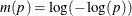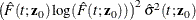### Proportional Subdistribution Hazards Model for Competing-Risks Data

Subsections:

Competing risks arise in the analysis of time-to-event data when the event of interest can be impeded by a prior event of a different type. For example, a leukemia patient’s relapse might be unobservable because the patient dies before relapse is diagnosed. In the presence of competing risks, the Kaplan-Meier method of estimating the survivor function is biased, because you can no longer assume that a subject will experience the event of interest if the follow-up period is long enough. The cumulative incidence function (CIF), which is the marginal failure subdistribution of a given cause, is widely used in competing-risks analysis.

The proportional hazards model for the subdistribution that Fine and Gray (1999) propose aims at modeling the cumulative incidence of an event of interest. They define a subdistribution hazard,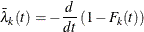where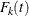is the cumulative incidence function for the failure of cause k, and they impose a proportional hazards assumption on the subdistribution hazards: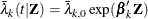The estimation of the regression coefficients is based on modified risk sets, where subjects that experience a competing event are retained after their event. The weight of those subjects that are artificially retained in the risk sets is gradually reduced according to the conditional probability of being under follow-up had the competing event not occurred.

You use PROC PHREG to fit the Fine and Gray (1999) model by specifying the EVENTCODE= option in the MODEL statement to indicate the event of interest. Maximum likelihood estimates of the regression coefficients are obtained by the Newton-Raphson algorithm. The covariance matrix of the parameter estimator is computed as a sandwich estimate. You can request the CIF curves for a given set of covariates by using the BASELINE statement. The PLOTS=CIF option in the PROC PHREG statement displays a plot of the curves. You can obtain Schoenfeld residuals and score residuals by using the OUTPUT statement.

To model the subdistribution hazards for clustered data (Zhou et al., 2012), you use the COVS(AGGREGATE) option in the PROC PHREG statement. You also have to specify the ID statement to identify the clusters. To model the subdistribution hazards for stratified data (Zhou et al., 2011), you use the STRATA statement. PROC PHREG handles only regular stratified data that have a small number of large subject groups.

When you specify the EVENTCODE= option in the MODEL statement, the ASSESS, BAYES, and RANDOM statements are ignored. The ATRISK and COVM options in the PROC PHREG statement are also ignored, as are the following options in the MODEL statement: BEST=, DETAILS, HIERARCHY=, INCLUDE=, NOFIT, PLCONV=, RISKLIMITS=PL, SELECTION=, SEQUENTIAL, SLENTRY=, SLSTAY=, TYPE1, and TYPE3(LR, SCORE). Profile likelihood confidence intervals for the hazard ratios are not available for the Fine and Gray competing-risks analysis.

#### Parameter Estimation

For the ith subject,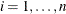, let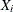,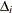,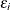, and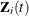be the observed time, event indicator, cause of failure, and covariate vector at time t, respectively. Assume that K causes of failure are observable (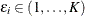). Consider failure from cause 1 to be the failure of interest, with failures of other causes as competing events. Let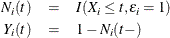Note that if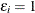, then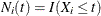and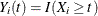; if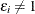, then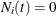and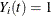. Let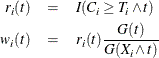where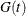is the Kaplan-Meier estimate of the survivor function of the censoring variable, which is calculated using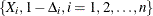. If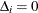, then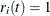when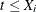and 0 otherwise; and if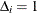, then. Table 71.12 displays the weight of a subject at a function of time.

Table 71.12: Weight for the ith Subject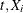Status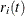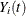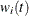1 1 1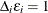1 1 1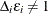1 1 1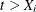0 1 01 0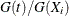1 1The regression coefficientsare estimated by maximizing the pseudo-likelihood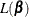with respect to: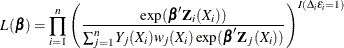The variance-covariance matrix of the maximum likelihood estimator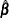is approximated by a sandwich estimate.

With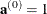,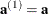, and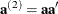, let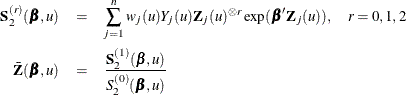The score function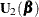and the observed information matrix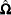are given by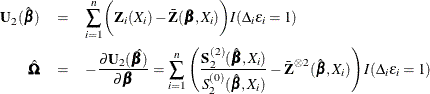The sandwich variance estimate ofis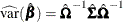where
is the estimate of the variance-covariance matrix of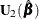that is given by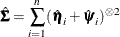where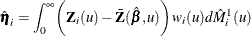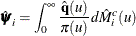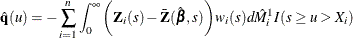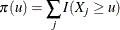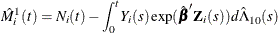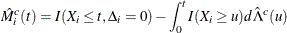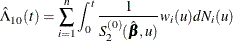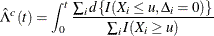#### Residuals

You can use the OUTPUT statement to output Schoenfeld residuals and score residuals to a SAS data set.

 Schoenfeld residuals: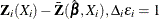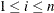Score residuals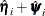#### Cumulative Incidence Prediction

For an individual with covariates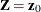, the cumulative subdistribution hazard is estimated by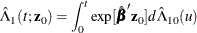and the predicted cumulative incidence is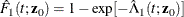To compute the confidence interval for the cumulative incidence, consider a monotone transformation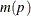with first derivative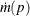. Fine and Gray (1999, Section 5) give the following procedure to calculate pointwise confidence intervals. First, you generate B samples of normal random deviates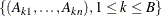. You can specify the value of B by using the NORMALSAMPLE= option in the BASELINE statement. Then, you compute the estimate of var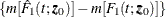as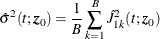where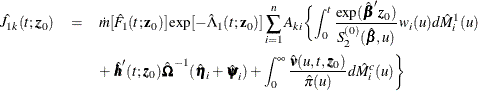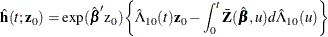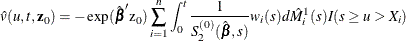A 100(1–)% confidence interval for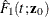is given by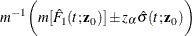where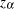is the 100(1–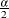) percentile of a standard normal distribution.

The CLTYPE option in the BASELINE statement enables you to choose the LOG transformation, the LOGLOG (log of negative log) transformation, or the IDENTITY transformation. You can also output the standard error of the cumulative incidence, which is approximated by the delta method as follows: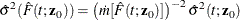Table 71.13 displays the variance estimator for each transformation that is available in PROC PHREG.

Table 71.13: Variance Estimate of the CIF Predictor

 CLTYPE= keyword Transformation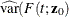IDENTITY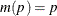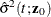LOG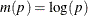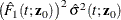LOGLOG